Games
Problems
Go Pro!

# Physics

Reference > Science > Physics

# Featured Reference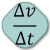Motion in one dimension. Acceleration, velocity, displacement explanations, and many word problems. Unit 1 of the Physics Study Guide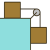Dynamics - Causes of motion and Newton's Laws of motion. Includes many problems to solve. Unit 2 of the Physics Study Guide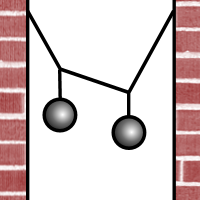Physical quantities used in physics with direction and size. Examples: displacement, velocity, acceleration, force, torque, momentum, angular momentum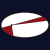Kinematics unit on motion in curved lines - projectile motion, uniform circular motion, and orbital motion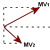Impulse and Momentum unit, including a section of collision problems and solutions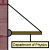Rotational Motion and Simple Harmonic Motion - explanations, sample problems, and practice problems. Unit Six of the Study Guide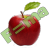Sir Isaac Newton dramatically increased our understanding of the universe with his laws of motion and gravitation.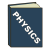Various appendices to the Physics Study Guide written by Mr. Roger Twitchell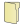# Full Directory Listing

Newton's LawsLike us on Facebook to get updates about new resources